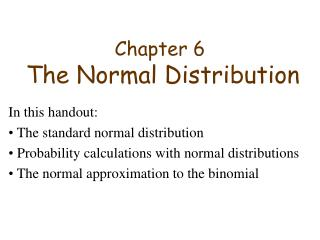Download PresentationChapter 6 The Normal Distribution

# Chapter 6 The Normal Distribution - PowerPoint PPT Presentation

Chapter 6 The Normal Distribution. In this handout: The standard normal distribution Probability calculations with normal distributions The normal approximation to the binomial. Figure 6.8 (p. 231) The standard normal curve. It is customary to denote the standard normal variable by Z.I am the owner, or an agent authorized to act on behalf of the owner, of the copyrighted work described.
Download Presentation## Chapter 6 The Normal Distribution

An Image/Link below is provided (as is) to download presentation

Download Policy: Content on the Website is provided to you AS IS for your information and personal use and may not be sold / licensed / shared on other websites without getting consent from its author.While downloading, if for some reason you are not able to download a presentation, the publisher may have deleted the file from their server.

- - - - - - - - - - - - - - - - - - - - - - - - - - E N D - - - - - - - - - - - - - - - - - - - - - - - - - -
Presentation Transcript
1. Chapter 6The Normal Distribution In this handout: The standard normal distribution Probability calculations with normal distributions The normal approximation to the binomial

2. Figure 6.8 (p. 231)The standard normal curve. It is customary to denote the standard normal variable by Z. Figure 6.9 (p. 232)Equal normal tail probabilities.

3. From the table, P[Z ≤ 1.37] = .9147 An upper tail normal probability P[Z > 1.37] = 1 - P[Z ≤ 1.37] = 1 - .9147 = .0853

4. Figure 6.11 (p. 233)Normal probability of an interval. P[-.155 < Z < 1.6] = P[Z < 1.6] - P[Z < -.155] = .9452 - .4384 = .5068

5. Figure 6.12 (p. 233)Normal probabilities for Example 3. P[Z < -1.9 or Z > 2.1] = P[Z < -1.9] + P[Z > 2.1] = .0287 + .0179 = .0466

6. Determining an upper percentile of the standard normal distribution • Problem: Locate the value of z that satisfies P[Z > z] = 0.025 • Solution: P[Z < z] = 1- P[Z> z] = 1- 0.025 = 0.975 From the table, 0.975 = P[Z < 1.96]. Thus, z = 1.96

7. Determining z for given equal tail areas • Problem: Obtain the value of z for which P[-z < Z < z] = 0.9 • Solution: From the symmetry of the curve, P[Z < -z] = P[Z > z] = .05 From the table, P[Z < -1.645] = 0.05. Thus z = 1.645

8. Converting a normal probability to a standard normal probability (example) • Problem: Given X is N(60,4), find P[55 < X < 63] • Solution: The standardized variable is Z = (X – 60)/4 x=55 gives z=(55-60)/4 = -1.25 x=63 gives z=(63-60)/4 = .75 Thus, P[55<X<63] = P[-1.25 < Z < .75] = P[Z < .75] - P[Z < -1.25] = .7734 - .1056 = .6678

9. When the success probability p of is not too near 0 or 1 and the number of trials is large, the normal distribution serves as a good approximation to the binomial probabilities. Figure 6.16 (p. 241)The binomial distributions for p = .4 and n = 5, 12, 25.

10. How to approximate the binomial probability by a normal? The normal probability assigned to a single value x is zero. However, the probability assigned to the interval x-0.5 to x+0.5 is the appropriate comparison (see figure). The addition and subtraction of 0.5 is called the continuity correction.

11. Example: Suppose n=15 and p=.4. Compute P[X = 7]. Mean = np = 15 * .4 = 6 Variance = np(1-p) = 6 * .6 = 3.6 sd = 1.897 P[6.5 < X < 7.5] = P[(6.5 -6)/1.897 < Z < (7.5 - 6)/1.897] = P[.264 < Z < .791] = .7855 - .6041 = .1814